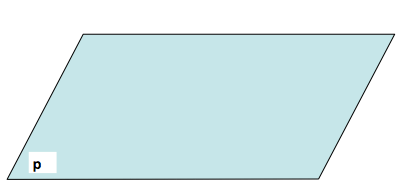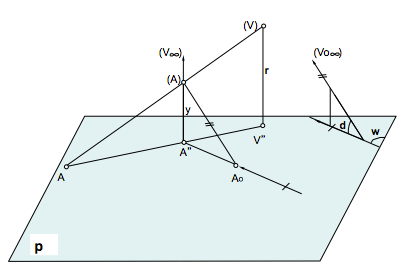# Representation systems : Projections [ Descriptive geometry ]Called Representation Systems encompass a set of techniques and projection models elements for viewing three-dimensional space on a two dimensional plane.

Each of the systems provides a number of advantages that make it particularly useful in certain applications. So, systems that are included in the overall perspectives, are especially useful to give a simple three-dimensional view of the object. Cylindrical nature systems facilitate operations orthogonal measure to reduce them to obtain Pythagorean triangles (rectangles), models while central conical or approximate how the human eye works.

The geometry descriptive is a set of techniques that can represent geometric character dimensional space on a two dimensional surface and, therefore, solving two-dimensional spatial problems ensuring the reversibility of the process through the proper reading.(W)

All systems can be studied from a projective point of view by the two fundamental operations: Projection and section. Some aspects as those concerning the incidence or membership may become independent of the projection model used, so it can be addressed in a general.

These latter notions lead us to relate the different systems into a single figure at the time of initiating the study, providing a performance space projective fundamental notions while bridges establish methodological including.

Conical Perspective, Diédrico System, Axonometric Perspective and Perspective are Caballera Systems using conical projection procedures, orthogonal and oblique, which may be linked in a shape that provides for co.

1º) Consider a projection plane, drawing plane, plane of the paper or picture plane, which for brevity called p.2º) The three vertices of projection orthogonal, oblique conical will correspond to the three basic projection leading to different representation systems famílias.3º) Be a point (A), representation object. Let's see how is projected onto the projection plane from each of the vertices or projection centers mentioned.4º) Making your first performance in orthogonal projection. The projection of the point on the plane is the intersection of the beam projecting to the projection plane, namely, the line containing the point and the center of projection.5º) Also projected (A) oblique cone-shaped from the corresponding projection centers.6º) In conical projection two right triangles are similar and are similar oblique projection two
The first angle triangles share g, the second angle d and one of the first one of the second leg and7º) When considering any straight line passing through (A), to is the conic projection, a” orthogonal to oblique.

8º) Three coincide with the intersection point the projection plane.9º) Therefore a-a” its perspectives center In ", a”-ao I am with center V.. and a-ao center Within
10º) A center perspective always involves improper conservation associated simple reason.
11ª) With the center itself is not preserved the simple reason. but double reason.
12º) Angle to the straight is determined in a right triangle with legs a” and and.Later establish the geometric condition that distinguishes the orthogonal projection to the oblique projection (respect to the conical projection is also iterable), will be discussed in the so-called theorem of three perpendicular.

Acknowledgements: The teacher Jaime Rua José Armesto by its sequence of images and comments on the subject.